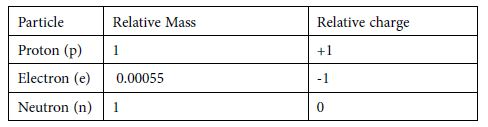Chemistry

# Fundamental Subatomic Particles

Electrons are only found outside the nucleus. We can say that the electron has a negligible mass because it is —2000 times less heavy than both the proton and neutron. The atom is neutral therefore the number of protons is equal to the number of electrons which in turn is equal to the atomic number of atom. The Atomic Number is the number of protons (equal to the number of electrons). The Mass Number is the number of protons + neutrons. Neutrons do not change the way that an element behaves chemically.Different number of protons and electrons makes a different element; one which behaves in a different way chemically. Elements are typically represented in the following way: chemically. Elements are typically represented in the following way:

AXZ

Where A is the Mass Number, Z the Atomic Number and X the symbol.

E.g.: 23Na11 This sodium atom contains 11 protons, 11 electrons and 23 – 11 = 12 neutrons 11.